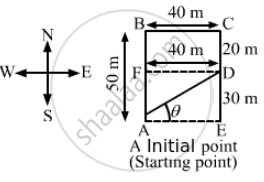Advertisement Remove all ads

# A Man Has to Go 50 M Due North, 40 M Due East and 20 M Due South to Reach a Field. (A) What Distance He Has to Walk to Reach the Field? (B) What is His Displacement from His House to the Field? - Physics

Sum

A man has to go 50 m due north, 40 m due east and 20 m due south to reach a field. (a) What distance he has to walk to reach the field? (b) What is his displacement from his house to the field?

Advertisement Remove all ads

#### Solution(a) Distance travelled by the man = AB + BC + CD = 50 + 40 + 20 = 110 m
(b) AF = AB − BF = 50 − 20 = 30 m
Displacement = Final position − Initial position = AD

$\therefore AD = \sqrt{{AF}^2 + {DF}^2} = \sqrt{{30}^2 + {40}^2}$

$= 50 \text{ m }$

In ∆AED,

$\tan \theta = \frac{DE}{AE} = \frac{30}{40}$
$\Rightarrow \theta = \tan^{- 1} \left( \frac{3}{4} \right)$

Displacement from the house to the field = 50 m in the direction $\tan^{- 1} \left( \frac{3}{4} \right)$ north to east.

Is there an error in this question or solution?
Advertisement Remove all ads

#### APPEARS IN

HC Verma Class 11, 12 Concepts of Physics 1
Chapter 3 Rest and Motion: Kinematics
Q 1 | Page 51
Advertisement Remove all ads

#### Video TutorialsVIEW ALL 

Advertisement Remove all ads
Share
Notifications

View all notifications

Forgot password?